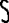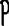×

×

USC / Engineering / Chem 111 / What mass of o2 will react with 5.00 g of h2?

# What mass of o2 will react with 5.00 g of h2? Description

##### Description: These notes cover important definitions, rules, and some practice problems for the exam on 2/15.
8 Pages 61 Views 2 Unlocks
Reviews

zacharytweeks.1 (Rating: )

Definitions

## What mass of o2 will react with 5.00 g of h2?Substances: a material that is chemically the same

Mixtures: matter that can be separated into simpler materials by physical means Accuracy: the agreement of a set of measures with the true value

Precision: the agreement among repeated measurements of the same quantity (results that are  similar to each other)

Number of significant figures: the number of digits from the first non­zero digit through the last  reported digit

Atom: the smallest unit

Element: composed entirely of one atom

Compound: two or more different elements

Law of Conservation of Mass: atoms do not change mass or density when a chemical reaction  occurs

## What are the oxidation numbers for p and o in po4 3­?Atomic Number (Z) is the number of protons in an element

Mass Number (A) is the sum of protons and neutrons

Cation: has a positive charge and forms when an atom loses one or more electron Anion: has a negative charge and forms when an atom gains one more electron Isotopic mass: the mass, in u, of a particular isotope of an element

Atomic mass: the weighted average mass of the naturally occurring isotopes of an element Molecular mass: the sum of all the atomic masses in a molecule

Solubility Rules

Soluble compounds:

Group 1A cation and NH4+

Nitrates (NO3­)

## What are the oxidation numbers for k, s, and o in k2so4?Perchlorates (ClO4­)

Acetates (CH3COO­)

Chlorides, Bromides, Iodides (Cl­, Br­, I­)  If you want to learn more check out How do you calculate turbine efficiency?

Except with! (Ag+, Hg22+, Pb2+)

Sulfates (SO42­)

Except with! (Sr2+, Hg22+, Pb2+, Ba2+) We also discuss several other topics like What are the main reason why sexed semen is important?

Insoluble compounds:

Carbonates (CO32­)

Except with! Group 1A cations and NH4+

Phosphates (PO43­)

Except with! Group 1A cations and NH4+

Hydroxides (OH­)

Except with! Group 1A cations and NH4+, Sr2+, and Ba2+

Rules for Assigning Oxidation Numbers (IMPORTANT)

∙ An atom in its elemental state has an oxygenic number of zero.

∙ The oxygenic number of monatomic ions is the charge of the ion.

∙ In combination with other elements, the oxidation number of F is ­1, O is generally ­2, H

is generally +1 with a nonmetal and ­1 with a metal. Other halogens are generally ­1.  ∙ The sum of the oxidation numbers in any species must equal the charge of the species.  We also discuss several other topics like What comes out of volcanoes?

Common Polyatomic Anions to Memorize

Carbonate CO32­        Phosphate PO43­                   Hydroxide OH Nitrate NO3­ Sulfate SO42­  Nitrate No3­ If you want to learn more check out How do you find the probability distribution?

Chlorate CO32­ Sulfite SO42­

1a 2a

Transition

Metals

Lanthanides

Actinides

3a 4a 5a

 1a  alkali metals: soft, reactive, metals   2a  alkaline metals

 7a  halogens: reactive nonmetals

 8a  noble gases: the stable elements

Conversions and Formulas

1mL = 1 cm3

TF = Tc x (1.8°F/1.0°C) +32°F

TC = (TF ­ 32°F) x (1.0°C/1.8°F)

TK = TC + 273.15

Density = Mass/Volume

Mass percentage = (mass of element/ mass of compound) * 100

Practice Examples

~Calculate the mass, in grams, of Al(OH)3 (molar mass = 78) formed by the reaction of exactly 0.500 L of 0.100  M NaOH with excess Al(NO3)3 Don't forget about the age old question of What is the function of pericardium?

Solution:

1) Write and balance equation

a. Al(NO3)3 (aq) + 3NaOH  3NaNO3(aq)  + Al(OH)3(s)

2) Use M = mol/L to find moles/liters of what you are given

a. 0.100 M = (mol/0.500 L) Don't forget about the age old question of What happens when thyroid stimulating hormone is high?

= 0.0500 mol NaOH

3) Use the coefficients in the equation to convert from one compound to the other a. 0.500 mol NaOH (1mol Al(OH)3/3 mol NaOH) = 0.167 mol Al(OH)3 4) Convert moles to grams of desired compound

a. 0.167 mol Al(OH)3  (78.0 g Al(OH)3/ 1 mol Al(OH)3) = 1.30 g Al(OH)3

Which symbol listed below describes a particle with 15 protons, 16 neutrons, and 16 electrons?

A. B. C. D.Solution: E

~What mass of O2 will react with 5.00 g of H2? (Based on the give balanced equation) 2H2 + O2  2H20

Solution:

1) Start with mass given in the question and convert that to moles

a. 5.00 g H2 (1 mol H2/2.02 g H2) = 2.50 mol H2

2) Next use the balanced equation to convert to moles of O2 (use the coefficients in the

equation)

a. 2.50 mol H2 (1 mol O2/2 mol H2) = 1.25 mol O2

3) Now convert moles of O2 to mass (use periodic table to get mass of O2) a. 1.25 mol O2 (32.0 g O2/1 mol O2) = 40.0 g O2

~Predict the solubility of the following compounds:

1) NH4OH

2) FeSO4

3) PbCl2

4) Ca(NO3)2

5) K2CO3

Solution:

1) soluble

2) soluble

3) insoluble

4) soluble

5) soluble

~What are the oxidation numbers for P and O in PO43­?

Solution:

O: ­2

P: +5

We know that P is +5 because we know that O is ­2 and there are 4 of them so (­2) x 4 =  8. P must be +5 in order to give a 3­ charge overall.

~What are the oxidation numbers for K, S, and O in K2SO4?

Solution:

O: ­2

S: +6

K: +1

Since we know that O is ­2, we start there. We also know that K is +1 (from the rules). Since we  have ­2(4) = 8 for O and +1(2) = 2 for K, the S must be +6

Name the element: Na2S

a. sodium sulfate

b. sulfur iodide

c. sodium sulfide

d. sodium selenide

e. none of these

Solution: C

What is the formula of barium phosphate?

Ba2(PO3)3

Ba(PO4)2

Ba2PO4

Ba3(PO4)2

Solution: D

The formula mass of sodium sulfate is:

Na+  and SO42­

Na2SO4

2(Na) = 2 x 23

1 S = 32

4 O = 4 x 16

Total: 142 u

How many atoms are present in 0.35 mol of Na?

0.35 mol Na *   (6.022 x 1023/ 1 mol Na)

= 2.1 x 1023 atoms Na

How many moles are present in 3.00 x 1021 molecules of C2H6? 3.00 x 1021 C2H6 * (1 / 6.022 x 1023)

= 4.98 x 10 ­3 mols C2H6

Name the Molecules

1. XeF6

a. Xenon hexafluoride

2. N2O5

a. Dinitrogen pentoxide 3. Fe (OH)3

a. Iron (III) Hydroxide  4. HNO2

a. Nitrous acid

Page ExpiredIt looks like your free minutes have expired! Lucky for you we have all the content you need, just sign up here